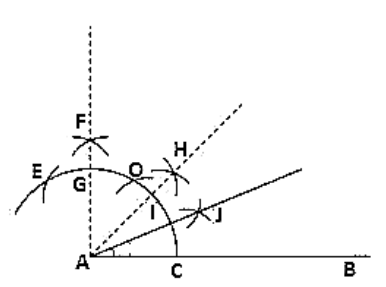# Construct the angles of the following measurements:

Question:

Construct the angles of the following measurements:

(i) 30°

(ii) 75°

(iii) 105°

(iv) 135°

(v) 15°

(vi) 22(1/2)°

Solution:

(i) Steps of construction:

1. Draw a line segment AB.

2. Keeping A as the centre and any radius draw an arc which intersects AB at C.

3. Keeping C as center and the same radius draw an arc which intersects the previous arc at D.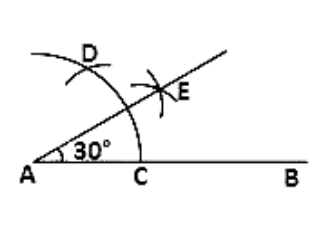(ii) Steps of construction:

1. Draw a line segment AB

2. Keeping A as center and any radius draw an arc which intersects AB at C

3. Keeping C as center and the same radius draw an arc which intersects the previous arc at D

4. Keeping D as center and same radius draw an arc which intersects arc in (2) at E

5. Keeping E and D as center and radius more than half of ED, draw arcs intersecting each other at F

6. Join FA which intersects arc in (2) at G

7. Keeping G and D as center and radius more than half of GD draw arcs intersecting each other at H

8. Join HA

Therefore ∠HAB = 75°

4. Keeping D and C as center and radius more than half of DC draw arcs which intersect each other at E.

5. Join EA.

Therefore ∠EAB = 30°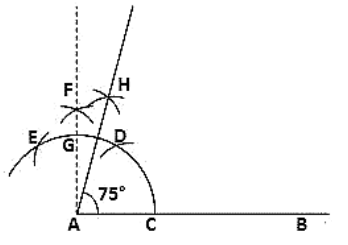(iii) Steps of construction:

1. Draw a line segment AB.

2. Keeping A as the center and any radius draw an arc which intersects AB at C.

3. Keeping C as center and the same radius draw an arc which intersects the previous arc at D.

4. Keeping D as the centre and same radius draw an arc which intersects arc in (2) at E.

5. Keeping E and D as center and radius more than half of ED draw arcs which intersect each other at F.

6. Join FA which intersects arc in (2) at G.

7. Keeping E and G as center and radius more than half of EG draw arcs which intersect each other at H.

8. Join HA.

Therefore ∠HAB = 105°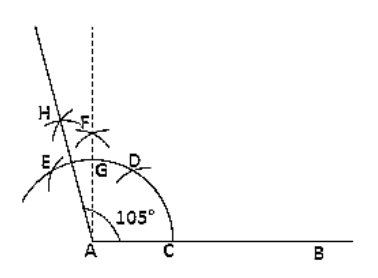(iv)  Steps of construction:

1. Draw a line segment AB and produce BA to C.

2. Keeping A as the center and any radius draw an arc which intersects AC at D and AB at E.

3. Keeping D and E as center and radius more than half of DE draw arcs which intersect each other at F.

4. Join FA which intersects the arc in (2) at G.

5. Keeping G and D as center and radius more than half of GD draw arcs which intersect each other at H

6. Join HA.

Therefore ∠HAB = 135°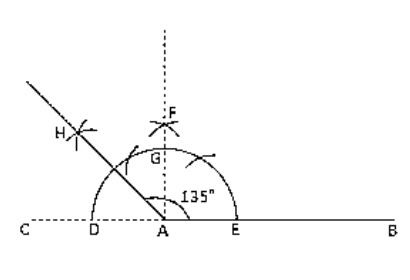(v) Steps of construction:

1. Draw a line segment AB.

2. Keeping A as the centre and any radius draw an arc which intersects AB at C.

3. Keeping C as center and the same radius draw an arc which intersects the previous arc at D.

4. Keeping D and C as center and radius more than half of DC draw arcs which intersect each other at E.

5. Join EA which intersects arc in (2) at F.

6. Keeping F and C as center and radius more than half of FC draw arcs which intersect each other at G.

7. Join GA.

Therefore ∠GAB = 15°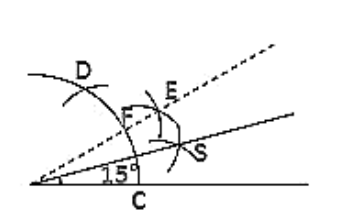(vi) Steps of construction:

1. Draw a line segment AB.

2. Keeping A as the center and any radius draw an arc which intersects AB at C.

3. Keeping C as center and the same radius draw an arc which intersects the previous arc at D.

4. Keeping D as the centre and same radius draw an arc which intersects arc in (2) at E.

5. Keeping E and D as center and radius more than half of ED draw arcs which intersect each at F.

6. Join FA which intersects arc in (2) at G.

7. Keeping G and C as center and radius more than half of GC draw arcs intersecting each other at point H.

8. Join HA which intersects the arc in (2) at a point I.

9. Keeping I and C as center and radius more than half of IC draw arcs intersecting each other at point J.

10. Join JA.

Therefore ∠JAB = 22(1/2)°